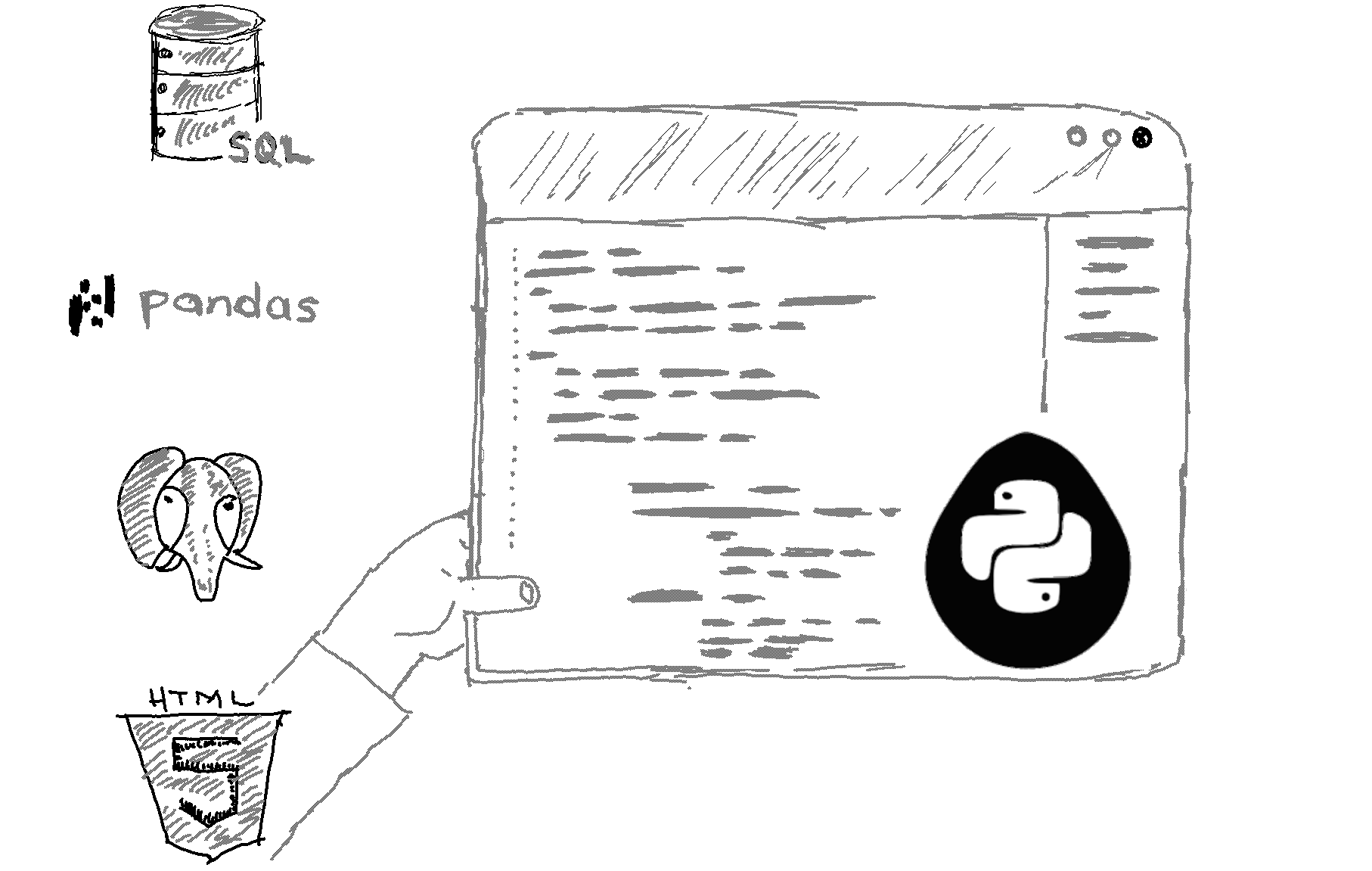# Here is how to check if a string is empty in Python.

```my_string = ""
if not my_string:
print("String is empty.") ```

Output

The 'not' operator returns True if the string is empty and False if it is not.

Explanation

In Python, you can check if a string is empty by using the 'not' operator as shown in the code above.

You can also use the 'len' function that returns the number of characters in a string. If the number of characters is 0, then the string is empty.

```my_string = ""
if len(my_string) == 0:
print("String is empty.")```
Another alternative would be to use the bool() function. This will check if the string is empty or not, returning True if it's empty and False if it's not.

```my_string = ""
if not bool(my_string):
print("String is empty.")```

Related HowTos
##### Recommended Course

Learn Flask development and learn to build cool apps with our premium Python course on Udemy.